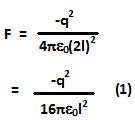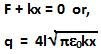## Tuesday, July 27, 2010

### Irodov Problem 3.54We shall use the Method of Images to solve this problem. Since this is the first problem that uses method of images, I will try and explain the idea behind Method of images first.

A perfect conductor is an equipotential surface. In other words there cannot be any potential difference between two points on a perfect conducting surface. Why is this so? Even the slighest potential difference along the surface of a perfect conductor will immideately result in a large current flow that will neutralize the potential difference. This is very similar to thermal conductors. You cannot sustain a temperature difference between any two points on a perfect thermal conductor since immideately heat will flow between the points until these two points are at the same temperature.

Equipotential surface in turn implies that there cannot be any electric field along the surface of the (tangential to the surface) of the conductor. In other words, in our problem, since the infinite conductor is along the x-y plane, Ex = Ey = 0.

If there were no infinite conducting plane, the electric field lines would move radially outwards from the charge q. However, the presence of the inifinite conducting plane distorts the electric field lines in such a manner that Ex = Ey = 0 at all points on the x-y plane. How does this happen? What really happens is that the electric field due to the charge q, causes the electrons in the conductor to move about and distribute themelves in such a manner that so as to make Ex = Ey = 0 all along the surface of the conductor. In other words, the charge q, induces a surface charge density distribution along the conductor's surface in a manner so as to ensure that Ex = Ey = 0 all along its surface.
One way to solve the problem is to determine the necessary charge distribution on the conductor's surface which results in Ex = Ey = 0 and then use this to solve the problem. A simpler way to solve the problem is using the "Method of Images". The idea is behind method of images is to replace the conducting surface by other imaginary charges in such a way that create the condition that tangential component of the electric field along the conducting surface is zero. This can be done by thinking of the conducting surface as a mirror. Imagine a person at the position of the charge q. What will he see? He will see another charge at the other side of the mirror that is at a distance equal to q's distance from the mirror. In method of images, each time you reflect a charge ithe sign of the charge is also negated (think of this as similar to lateral inversion phenomenon in mirror reflections). In other words we have an image charge of -q, on the other side of the conductor as shown in the figure.

Now let us examine how placing this imaginary charge helps us solve the problem. The electric filed lines between the charges q and -q are shown in the figure. The interesting thing to notice is that the field lines are perpendicular to the x-y plane at all points on the x-y plane. In other words Ex = Ey = 0 all over the infinite conducting plane. Thus, by placing the imaginary charge -q instead of the conducting plane we have created the same situation as it would have been if there were an infinite conducting plane. Now we can forget about the infinite conducting plane so solve the problem thinking that there is an imaginary image charge -q.

When the charge q is at a distance l from the conducting plane, the image charge -q is at a distance l from the conducting plane on the other side. The distance between the charges is thus 2l. The force F acting on the charge q is given by,Since the string is elongated by a length x, the restoring force acting on the charge due to the string is given by kx. Since the charge is static under the action of the two forces (there is no acceleration) we have,